# 你以为只是简单的排序？（二）## 归并排序

#### 归并排序思想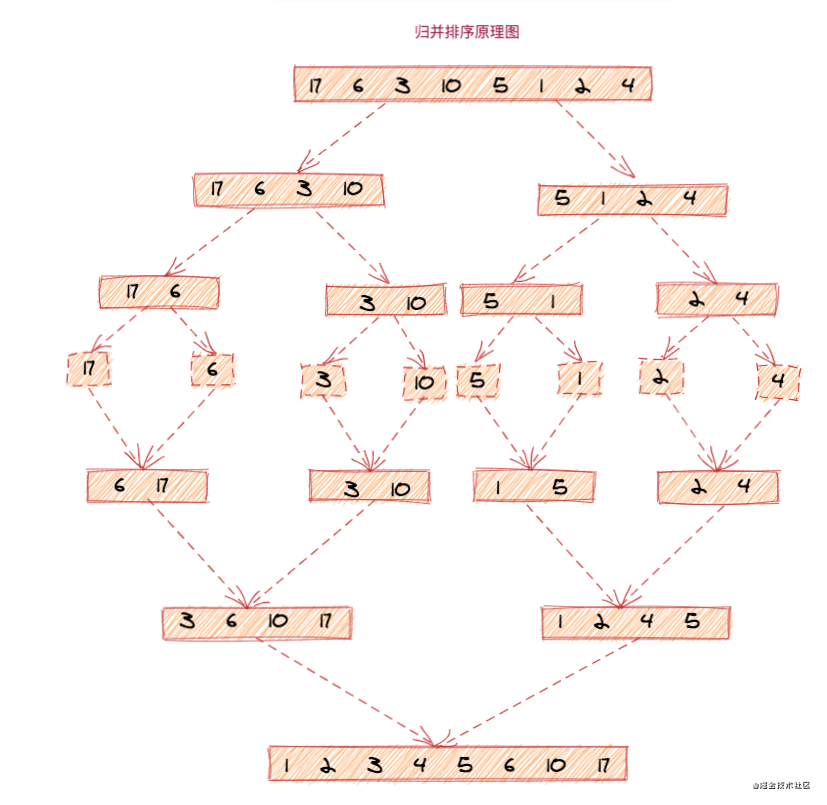﻿

#### 归并排序实现

递归公式MergeSort(start, end) = merge(MergeSort(start,(start+end)/2), MergeSort((start+end)/2 + 1, end))递归终止条件start >= end

MergeSort(start, end)表示的是给下标start到end之间的数组中的数据进行排序，将这个这个排序分成了两个子问题，一个是MergeSort(start,(start+end)/2)，另一个是MergeSort((start+end)/2 + 1), end)，当把这两个子问题排好序之后，再将它们合并，就把下标start到end之间的数据排好序了

﻿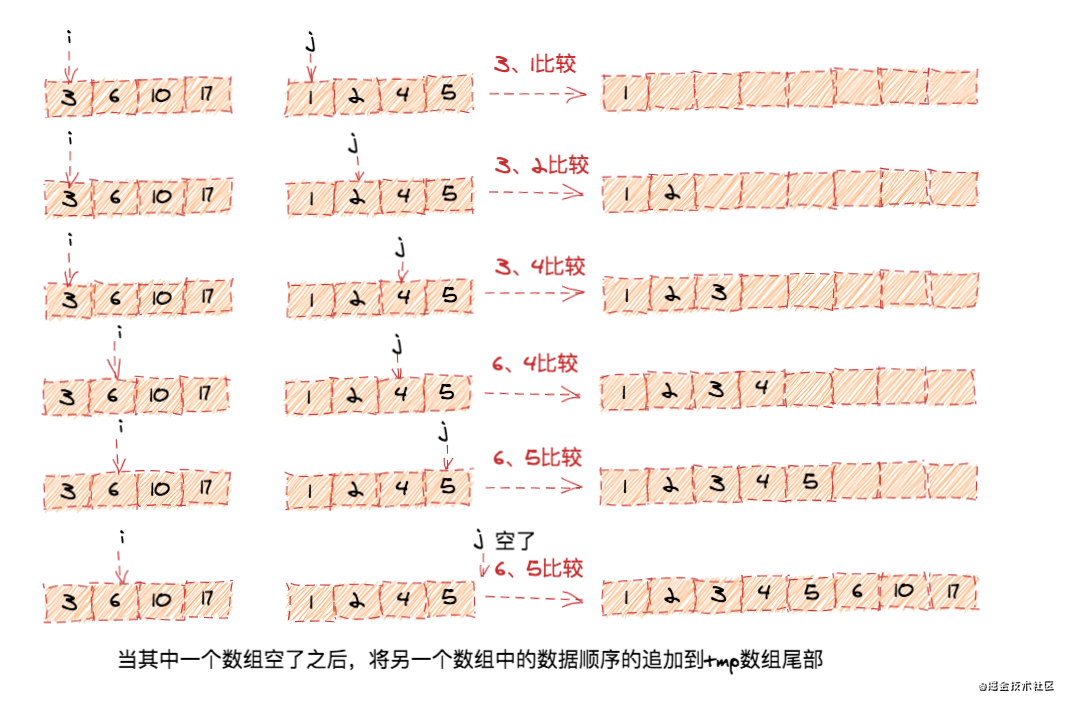func MergeSort(arr []int) []int {	if len(arr) <= 0 {		fmt.Println("参数不合法")		return nil	}	//递归终止条件，当拆分后的数组长度少于两个元素的时候	if len(arr) < 2 {		return arr	}	midIndex := len(arr) / 2	leftArr := MergeSort(arr[0:midIndex])	rightArr := MergeSort(arr[midIndex:])	result := merge(leftArr, rightArr)	return result}func merge(leftArr, rightArr []int) []int {	var mergeRes []int	leftIndex, rightIndex := 0, 0	leftLength, rightLength := len(leftArr), len(rightArr)	for leftIndex < leftLength && rightIndex < rightLength {		if leftArr[leftIndex] > rightArr[rightIndex] {			mergeRes = append(mergeRes, rightArr[rightIndex])			rightIndex++		} else {			mergeRes = append(mergeRes, leftArr[leftIndex])			leftIndex++		}	}	if leftIndex == leftLength{		for rightIndex < rightLength {			mergeRes = append(mergeRes, rightArr[rightIndex])			rightIndex++		}	}	if rightIndex == rightLength {		for leftIndex < leftLength {			mergeRes = append(mergeRes, leftArr[leftIndex])			leftIndex++		}	}	return mergeRes}

#### 归并排序性能分析

﻿

T(1) = C； n=1 时，只需要常量级的执行时间，所以表示为 C。T(n) = 2*T(n/2) + n； n>1

﻿

﻿

﻿

## 快速排序

#### 快速排序思想

﻿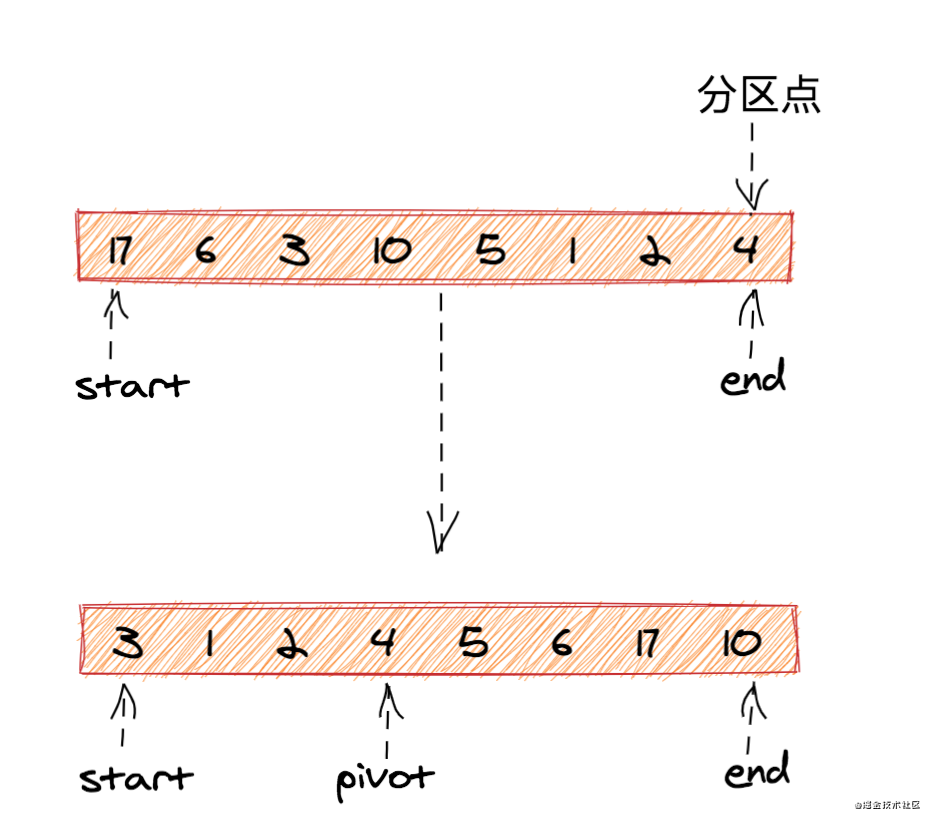﻿

﻿

公式：QuickSort(start...end) = QuickSort(start...pivot-1) + QuickSort(pivot+1...end)终止条件：start >= end

func QuickSort(arr []int, start int, end int) {  if start >= end {  	return  }  pivot := partition(arr, start, end)  QuickSort(arr, start, pivot-1)  QuickSort(arr, pivot+1, end)}

﻿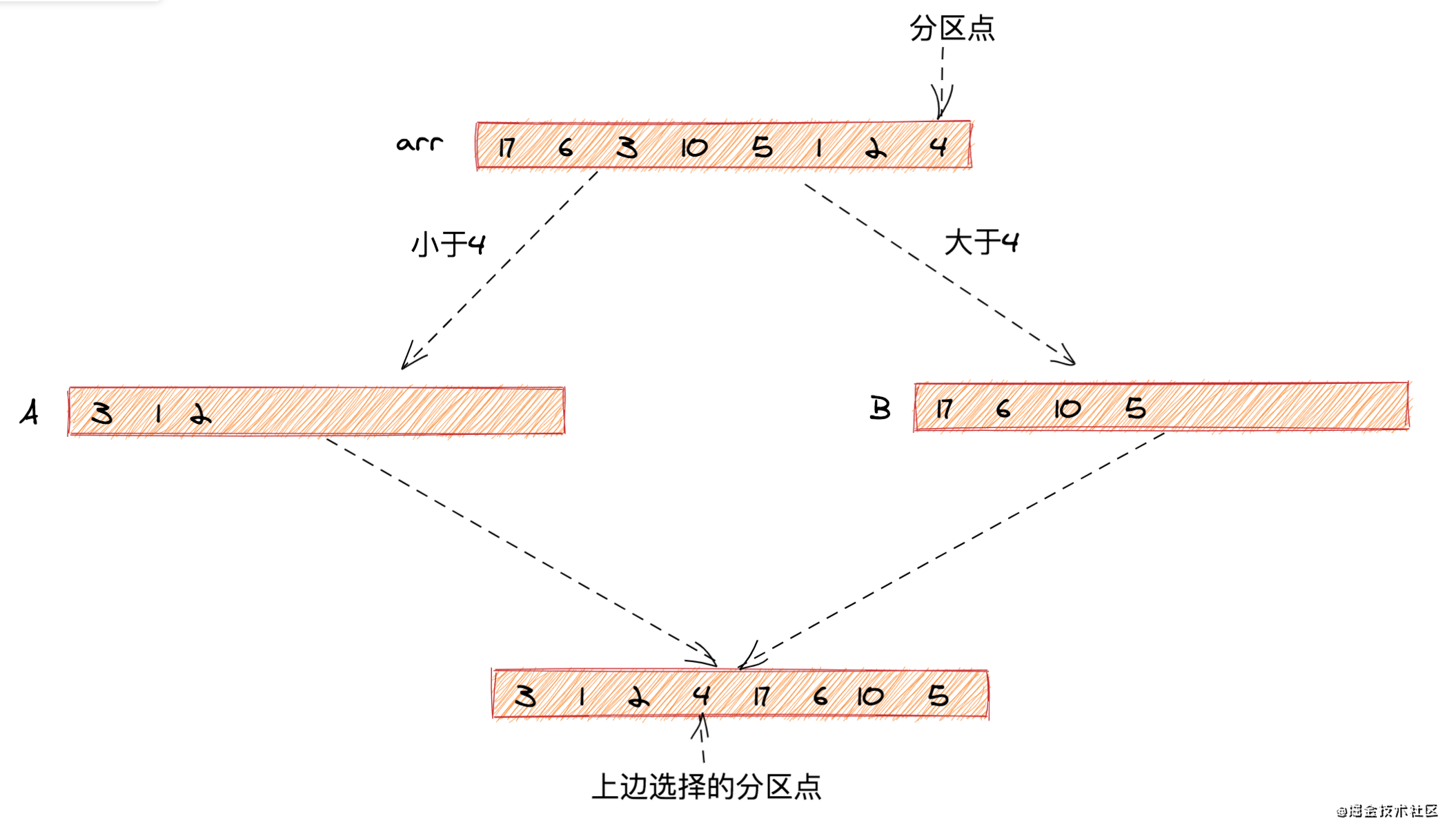﻿

func partition(arr []int, start int, end int) int {  pivotValue := arr[end]  i := start  for j:=start;j < end;j++ {    if arr[j] < pivot {      arr[i], arr[j] = arr[j],arr[i]      i++    }  }  arr[i], arr[end] = arr[end], arr[i]  return i}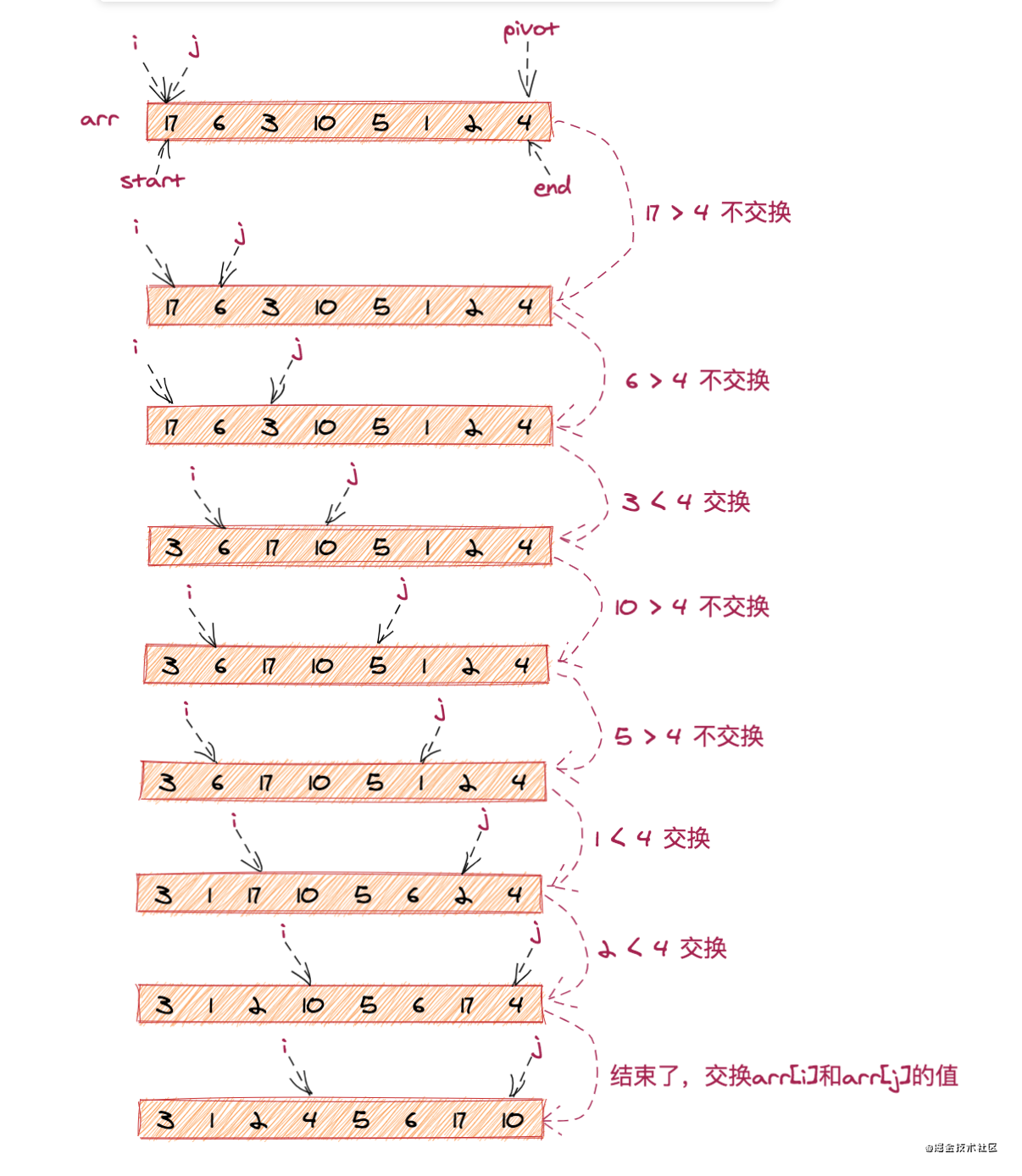#### 快速排序实现

package quickSortfunc QuickSort(arr []int, start int, end int) {  if start >= end {  	return  }  pivot := partition(arr, start, end)  QuickSort(arr, start, pivot-1)  QuickSort(arr, pivot+1, end)}func partition(arr []int, start int, end int) int {  pivotValue := arr[end]  i := start  for j:=start;j < end;j++ {    if arr[j] < pivotValue {      arr[i], arr[j] = arr[j],arr[i]      i++    }  }  arr[i], arr[end] = arr[end], arr[i]  return i}

#### 快速排序性能分析

﻿

﻿

T(1) = C； n=1 时，只需要常量级的执行时间，所以表示为 C。T(n) = 2*T(n/2) + n； n>1

﻿

## 归并排序和快速排序对比

#### 两种排序思想的区别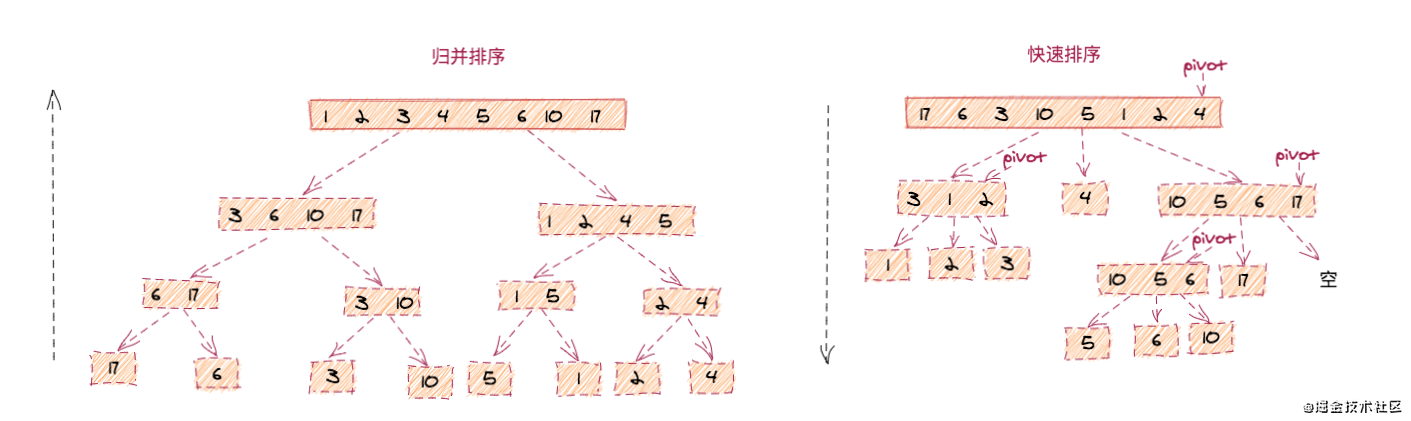#### 性能上的差异

﻿﻿## 评论